# Developing an AI Text Summarizer Using Python

## Developing an AI Text Summarizer Using Python:

Ever you feel like you don’t have extra time to read all the things that you want to? This blog “Developing an AI Text Summarizer Using Python” is going to help you in saving time whenever you have to go through a large complex paragraph.

In our normal routine, we all interact with applications that use text summarization apps. Some of these applications are for the platforms which publish articles on the daily news or entertainment.

Due to our hectic and busy schedules, we prefer to read a summary of the article to check its key phrases and main idea.

Reading a short summary gives us a brief context of the entire content and makes it easy for us to identify the interest area in a short time.

Text summarization is the process of generating a precise summary of any content without affecting its original meaning.

These online text summarizing tools use advanced NLP technology to summarize long content in less time.

• Why Do We Need To Build An AI Summarizer In Python?
• Approaches Used For The Text Summarization
• Developing an AI Text Summarizer By Using Python

## Why do we need to build an AI summarizer in Python?

Nowadays, whether it is an e-commerce platform or it is a public sector organization, everyone is mainly concerned to learn the customer’s feedback.

Consider, that these companies are getting hundreds of feedback every single day.

Therefore, it becomes a quite difficult task for them to analyze and manage the feedback and came up with accurate insights.

However, we noticed that advancements in technology can them to perform these tasks with less effort. The only thing that makes this happen is Machine Learning which has become capable of easily understanding the human language with NLP.

Right now, research is being made with the help of text analytics and one of its most recommended applications is a text summarizer.

The process of developing an AI summarizing tool in python helps users to shorten the feedback without affecting its original meaning.

This can be easily done by using an AI algorithm that reduces the text bodies while retaining their original meaning.

## Approaches used for the text summarization

In general, there are only two common types of summarizations i.e., abstractive and extractive. Both of these approaches help users to summarize text effectively and accurately.

### 1.    Abstractive Summarization

This method selects specific words based on semantic understanding, even if they didn’t appear in the source content.

The main focus of this summarization type is to generate important content in a whole new way. It examines the input content by using advanced NLP techniques to create a short version of the existing content.

That summarized text conveys the most important information from the actual content in a precise manner.

### 2.    Extractive Summarization

This summarizing type aims to summarize articles and other content by choosing a subset of specific words that retain the original meaning of the content.

This method of summarizing only focuses on key parts of the sentences to create a summary. It also uses AI to define weights for the sentences and prioritize them depending on their importance and similarity to each other.

Right now, there are a lot of summarizing tools have been developed in order to summarize text with either abstractive or extractive summarization approaches.

Furthermore, in this post, we are going to discuss these approaches help you to develop a summarizer in python.

## How to develop an AI Text Summarizer Using Python?

To develop an AI summarizing tool using python, follow the below steps:

### 1.    Launching a Google Colab Notebook

• Launch the notebook by first heading over to File > New Notebook.

### 2.    Install the required libraries

First, install the libraries by using the below code:

!pip install nltk

Import nltk

Now, import the libraries:

from nltk.corpus import stopwords

from nltk.cluster.util import cosine_distance

import numpy as np

import networkx as nx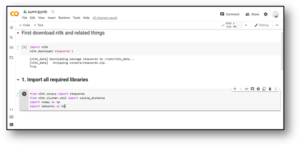### 3.    Generate clean sentences

Now, preprocess the text to clean the sentences and remove unnecessary words and notations in the text:

fil = open(fil_name, “r”)

artle = fildata.split(“. “)

sent = []   for sent1 in artle:

print(sent)

sent.append(sent1.replace(“[^a-zA-Z]”, ” “).split(” “))

sent.pop()

return sent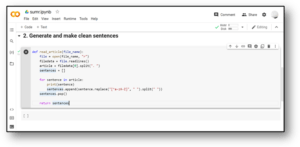### 4.    Check the sentence similarity

Now, use the cosine similarity to find similarities between sentence.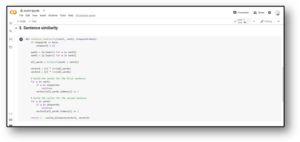### 5.    Built the matrix similarity

It’s important to grasp Cosine’s similarity in order to make the best use of the code you’ll encounter.

It measures the cosine of the angle between two non-zero vectors in an inner product space. Simply use it to identify sentence similarity while modeling the sentences as a collection of vectors. It computes the cosine of the angle between two vectors. If the phrases are similar, the angle will be 0.

def sim_matrix_func(sentences, stop_w):

smt = np.zeros((len(sentences), len(sentences)))

for idx1 in range(len(sentences)):

for idx2 in range(len(sentences)):

if idx1 == idx2: #ignore if both are same sentences

continue

smt[idx1][idx2] = sent_sim(sentences[idx1], sentences[idx2], stop_w)return smt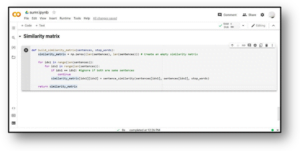### 6.    Generate the summary of the text

To keep the summarization pipeline running, continue to call the other auxiliary functions. Take a look at all of the steps in the code below:

def gen_summary_func(file_name, tp_h=3):

stop_words = stopwords.words(‘english’)

sum_txt = []    # Step 1

sent =  read_filefunc(file_name)    # Step 2 – Generate Sim Martix across the sentences

sent_sim_mtx = build_similarity_matrix(sent, stop_words)    # Step 3 – check the similarity martix

sent_sim_g = nx.from_numpy_array(sent_sim_mtx)

sc = nx.pagerank(sent_sim_g)    # Step 4: Select the best sentences

ran_sent = sorted(((scores[i],s) for i,s in enumerate(sent)), reverse=True)

print(“Indexes of best top ranked_sentences are “, ran_sent)for i in range(tp_h):

sum_txt.append(” “.join(ran_sent[i]))    # Step 5: Display the summarised text    print(“Summarize_Text is: \n”,  “.”.join(sum_txt))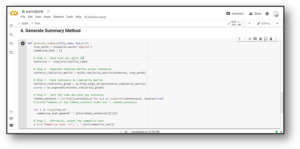### 7.    Finally, print the summary of the text

After that, print the summary by using the below code:

sumr = gen_summary_func(“newf.txt”, 2)

Print(sumr)## Conclusion

The process of developing an AI text summarizer in Python can be easily done by following the above steps. An AI-based summarizing tool maximizes your efficiency and minimizes the time required to get the main idea of the author.

Whether you are reading a notebook, article, or any academic document, the power of NLP and AI used in text summarizing tools will reduce the time you spend summarizing content manually.

We hope this article will help to easily understand the text summarization process and the sample overview of code to summarize the long content.